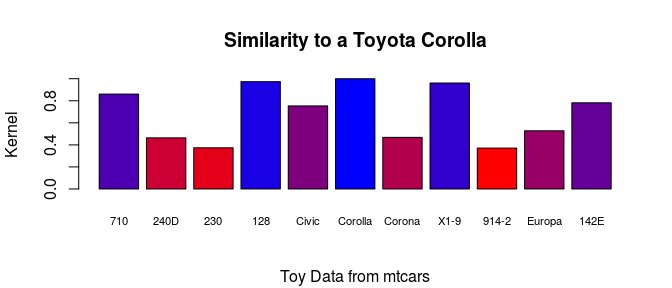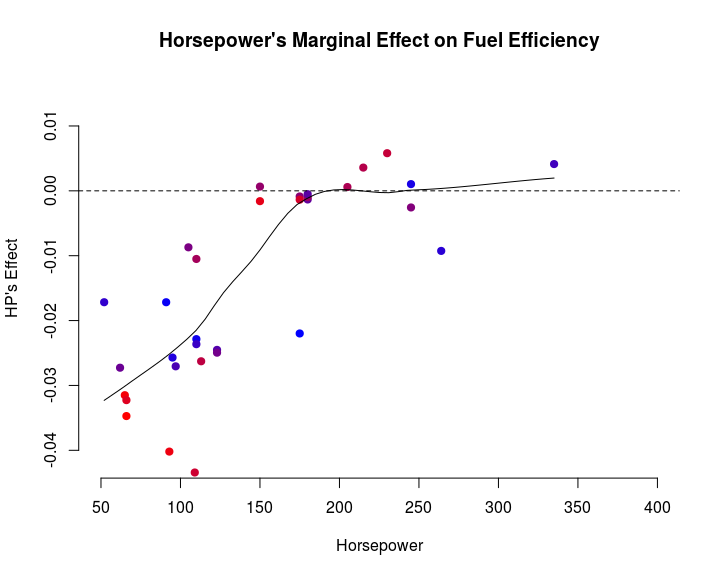# bigKRLS

Complex models are of increasing interest to social scientists. Researchers interested in prediction generally favor flexible, robust approaches, while those interested in causation are often interested in modeling nuanced treatment structures and confounding relationships. Unfortunately, estimators of complex models often scale poorly, especially if they seek to maintain interpretability.

Kernel Regularized Least Squares (KRLS) is a kernel-based, complexity-penalized method developed by Hainmueller and Hazlett (2013), which provides a good example of this kind of tradeoff. KRLS offers a desirable balance of interpretability, flexibility, and theoretical guarantees, primarily through the pointwise marginal derivative estimates produced by the estimation routine and their corresponding averages. However, these pointwise marginal derivatives are costly in time and memory to estimate.

Here, we introduce bigKRLS, an updated version of the original KRLS R package with algorithmic and implementation improvements designed to optimize speed and memory usage. These improvements allow users to straightforwardly fit KRLS models to medium and large data sets (N > ~2,500).

# Regression with bigKRLS

The bigKRLS() function is the workhorse of this package. There are only two basic inputs: a vector of N observations on the dependent variable, y, and an N x P matrix X, where P is the number of independent variables and also ncol(X).1

mtcars[1:5,]
##                    mpg cyl disp  hp drat    wt  qsec vs am gear carb
## Mazda RX4         21.0   6  160 110 3.90 2.620 16.46  0  1    4    4
## Mazda RX4 Wag     21.0   6  160 110 3.90 2.875 17.02  0  1    4    4
## Datsun 710        22.8   4  108  93 3.85 2.320 18.61  1  1    4    1
## Hornet 4 Drive    21.4   6  258 110 3.08 3.215 19.44  1  0    3    1
## Hornet Sportabout 18.7   8  360 175 3.15 3.440 17.02  0  0    3    2

Suppose we want to regress fuel efficiency on the other observables. Unlike classical regression, the KRLS algorithm does not directly estimate slope coefficients for particular variables. However, we can recover a similar set of quantities after estimation. In particular, the KRLS functional form allows users to calculate the marginal derivative dy/dxp at each observation, which can then be inspected or averaged to characterize the effect of each variable.

To estimate a model, use the bigKRLS() function:

reg.out <- bigKRLS(y = as.matrix(mtcars$mpg), X = as.matrix(mtcars[,-1]), Ncores = 1) ## .......................... ## All done. You may wish to use summary() for more detail, predict() for out-of-sample forecasts, or shiny.bigKRLS() to interact with results. For an alternative approach, see help(crossvalidate.bigKRLS). Type vignette("bigKRLS_basics") for sample syntax. Use save.bigKRLS() to store results and load.bigKRLS() to re-open them. Inspect the results using summary(): summary(reg.out) ## ## ## MODEL SUMMARY: ## ## lambda: 0.1306 ## N: 32 ## N Effective: 15.17858 ## R2: 0.9405 ## R2AME**: 0.8477 ## ## Average Marginal Effects: ## ## Estimate Std. Error t value Pr(>|t|) ## cyl -0.0733 0.0765 -0.9581 0.3806 ## disp -0.0037 0.0022 -1.6485 0.1582 ## hp -0.0145 0.0040 -3.5903 0.0148 ## drat 1.2786 0.6303 2.0287 0.0963 ## wt -1.4940 0.3115 -4.7968 0.0045 ## qsec 0.3678 0.1741 2.1123 0.0864 ## vs* 1.2772 0.6234 2.0488 0.0938 ## am* 0.9967 0.5954 1.6739 0.1530 ## gear 1.0847 0.2867 3.7828 0.0120 ## carb -0.5951 0.1518 -3.9200 0.0104 ## ## ## Percentiles of Marginal Effects: ## ## 5% 25% 50% 75% 95% ## cyl -0.3219 -0.1071 -0.0182 0.0065 0.0406 ## disp -0.0108 -0.0080 -0.0040 -0.0011 0.0044 ## hp -0.0372 -0.0258 -0.0138 -0.0009 0.0038 ## drat -0.4892 0.6973 1.1559 2.1076 3.4894 ## wt -3.5756 -1.9904 -1.2308 -0.7641 -0.2853 ## qsec -0.1250 0.0504 0.2099 0.5900 1.2423 ## vs* -0.3757 0.8754 1.2843 1.8167 2.6408 ## am* -0.6929 0.3432 0.8767 1.8618 2.7406 ## gear -0.4504 -0.1866 0.8420 1.9432 3.4149 ## carb -1.1902 -1.0159 -0.5844 -0.2336 0.0107 ## ## (*) Reported average and percentiles of dy/dx is for discrete change of the dummy variable from min to max (usually 0 to 1)). ## ## ## (**) Pseudo-R^2 computed using only the Average Marginal Effects. ## ## ## You may also wish to use predict() for out-of-sample forecasts or shiny.bigKRLS() to interact with results. Type vignette("bigKRLS_basics") for sample syntax. Use save.bigKRLS() to store results and load.bigKRLS() to re-open them. The model’s average marginal effect estimates (AMEs) can be interpreted similarly to the regression coefficients in a linear model, though estimates generated using the two methods may vary substantially depending on the level of effect heterogeneity present. The “Percentiles of the Marginal Effects” can be interpreted as evidence about whether y is a monotonic function of xp and the extent to which the effect of xp on y is homogeneous, if at all. In this toy data set, the number of cylinders is not a statistically significant predictor of fuel efficiency. Perhaps unsurprisingly, the marginal effect of cylinders is negative for about half of the cars investigated. By contrast, horsepower has a more uniformly negative effect on fuel efficiency. Suppose a user wanted to plot how similar a Toyota Corolla is to the other four cylinder cars: s <- reg.out$K[which(mtcars$cyl == 4), grep("Corolla", rownames(mtcars))] barplot(s, main = "Similarity to a Toyota Corolla", ylab = "Kernel", sub="Toy Data from mtcars", cex.names = .7, col = colorRampPalette(c("red", "blue"))(length(s))[rank(s)], names.arg = lapply(strsplit(rownames(mtcars), split=" "), function(x) x)[which(mtcars$cyl == 4)])Unsurprisingly, a Corolla is more similar to a Civic than a Porsche 914.

### Marginal effects

As noted above, fuel efficiency appears to be negatively related to horsepower. However, the effect of fuel efficiency on horsepower may not be monotonic:

scatter.smooth(mtcars$hp, reg.out$derivatives[,3], ylab="HP's Effect", xlab="Horsepower", pch = 19, bty = "n",
main="Horsepower's Marginal Effect on Fuel Efficiency",
col = colorRampPalette(c("blue", "red"))(nrow(mtcars))[rank(reg.out$coeffs^2)], ylim = c(-0.042, 0.015), xlim = c(50, 400)) abline(h=0, lty='dashed')The above graph suggests that, though lower-horsepower cars are generally more fuel-efficient, beyond a certain threshold that relationship weakens. ### Cross-Validation For users interested in out-of-sample predictive accuracy, bigKRLS() also provides a convenient crossvalidation function: CV.out <- crossvalidate.bigKRLS(y = as.matrix(mtcars$mpg), seed = 123, Kfolds = 4,
X = as.matrix(mtcars[,-1]), Ncores = 1)
## .......................
## ..........................
## ........................
## .............................
cor(CV.out$fold_3$tested$predicted, CV.out$fold_3$tested$ytest)
##  0.9736288

For fold 3, the predicted and actual values correlate at 0.97. To see a summary of the results, use summary():

summary(CV.out$fold_1$trained) # not run

CV.out contains several test statistics including:

CV.out$MSE_is ## fold1 fold2 fold3 fold4 ## 3.1302508 1.3776919 3.3185598 0.9130335 CV.out$MSE_oos
##    fold1    fold2    fold3    fold4
## 14.45841 11.09386 11.76474  8.41744
CV.out$R2_oos ## fold1 fold2 fold3 fold4 ## 0.7948766 0.6173782 0.9479531 0.7394939 CV.out$R2AME_oos
##     fold1     fold2     fold3     fold4
## 0.9176826 0.7238574 0.9445395 0.7125327

The first two test statatistics are the in- and out-of-sample mean squared error. The second two statistics show the performance of the full model (the N coefficients) compared with the portion that is linear and additive in X. To do a simple train and test, leave Kfolds blank and set ptesting instead.

### Shiny

To interact with your results in a pop up window or your browser, simply call:

shiny.bigKRLS(reg.out)         # not run

To remove the big square matrices so that you can easily put your results up on a server, use export:

shiny.bigKRLS(reg.out, export = T)         # not run

The output will describe the new, more compact object that has been created.

### Predicting with Out-of-Sample Data

Suppose a user wanted to know what percentage of cars would have lower gas mileage if they had 200 horsepower.

Xnew <- mtcars[,-1]
Xnew$hp <- 200 forecast <- predict(reg.out, as.matrix(Xnew)) ## . mean(forecast$predicted < mtcars\$mpg)
##  0.6875

Approximately 68.8% of cars would be less efficient in this scenario.

### “Big” File Management

When working with large datasets (N > 2,500), bigKRLS uses bigmemory objects for data management. As a result, calling save() and load() on a bigKRLS object will crash your R session. Instead you may do one of two things to save:

out <- bigKRLS(y, X, model_subfolder_name = "my_results") # not run
save.bigKRLS(out, "my_results") # not run

Either will save the model estimates to a new subfolder called “my_results” in your current working directory. To re-load:

load.bigKRLS("my_results") # not run

When N > 2,500, the bigKRLS() function will return most model outputs as bigmemory objects, which are really just memory addresses.

Z <- big.matrix(nrow=5, ncol=5, init=1)
Z
## An object of class "big.matrix"
## <pointer: 0x61376d0>
Z[]
##      [,1] [,2] [,3] [,4] [,5]
## [5,]    1    1    1    1    1